CAT  >  Practice Mock Test for SNAP - 14

# Practice Mock Test for SNAP - 14 - CAT

Test Description

## 60 Questions MCQ Test Mock Test Series for SNAP - Practice Mock Test for SNAP - 14

Practice Mock Test for SNAP - 14 for CAT 2023 is part of Mock Test Series for SNAP preparation. The Practice Mock Test for SNAP - 14 questions and answers have been prepared according to the CAT exam syllabus.The Practice Mock Test for SNAP - 14 MCQs are made for CAT 2023 Exam. Find important definitions, questions, notes, meanings, examples, exercises, MCQs and online tests for Practice Mock Test for SNAP - 14 below.
Solutions of Practice Mock Test for SNAP - 14 questions in English are available as part of our Mock Test Series for SNAP for CAT & Practice Mock Test for SNAP - 14 solutions in Hindi for Mock Test Series for SNAP course. Download more important topics, notes, lectures and mock test series for CAT Exam by signing up for free. Attempt Practice Mock Test for SNAP - 14 | 60 questions in 60 minutes | Mock test for CAT preparation | Free important questions MCQ to study Mock Test Series for SNAP for CAT Exam | Download free PDF with solutions
 1 Crore+ students have signed up on EduRev. Have you?
Practice Mock Test for SNAP - 14 - Question 1

### Spot the error part of the following sentences.

Detailed Solution for Practice Mock Test for SNAP - 14 - Question 1
Remove ‘more sweetly’ and use ‘sweeter’ in place of it.
Practice Mock Test for SNAP - 14 - Question 2

### Spot the error part of the following sentences.

Detailed Solution for Practice Mock Test for SNAP - 14 - Question 2
‘Hardly’ should be replaced by ‘hard’ as hardly shows negativity.
Practice Mock Test for SNAP - 14 - Question 3

### Spot the error part of the following sentences.

Detailed Solution for Practice Mock Test for SNAP - 14 - Question 3
Change ‘quite’ into ‘very’.
Practice Mock Test for SNAP - 14 - Question 4

Read the sentence to find out whether there is any grammatical error in it. The sentences are In three separate parts and each one is labelled (a), (b), (c) and (d). In that case, letter (d) will signify a ‘No error’ response.

Detailed Solution for Practice Mock Test for SNAP - 14 - Question 4

Change ‘an’ into ‘a’ because necessary starts with a consonant sound.

Practice Mock Test for SNAP - 14 - Question 5

Read sentence to find out whether there is any grammatical error in it. The sentences are In three separate parts and each one is labelled (a), (b), (c) and (d). In that case, letter (d) will signify a ‘No error’ response.

Detailed Solution for Practice Mock Test for SNAP - 14 - Question 5
Use ‘an’ in place of ‘one’.
Practice Mock Test for SNAP - 14 - Question 6

Find out the error part of the following sentences.

Detailed Solution for Practice Mock Test for SNAP - 14 - Question 6
Change ‘was living’ into ‘lived’ because simple past tense is here.
Practice Mock Test for SNAP - 14 - Question 7

Read sentence to find out whether there is any grammatical error in it. The sentences are In three separate parts and each one is labelled (a), (b), (c) and (d). In that case, letter (d) will signify a ‘No error’ response.

Detailed Solution for Practice Mock Test for SNAP - 14 - Question 7
Change ‘a’ into ‘the’ for historical building “The Taj Mahal”.
Practice Mock Test for SNAP - 14 - Question 8

Spot the error part of the following sentences.

Detailed Solution for Practice Mock Test for SNAP - 14 - Question 8
Remove ‘with’ before ‘tooth and nail’.
Practice Mock Test for SNAP - 14 - Question 9

Find out the error part of the following sentences.

Detailed Solution for Practice Mock Test for SNAP - 14 - Question 9
Use ‘has been’ in place of ‘is’ because we use Perfect Continuous Tense for a continued action of Past to Present.
Practice Mock Test for SNAP - 14 - Question 10

Read sentence to find out whether there is any grammatical error in it. The sentences are In three separate parts and each one is labelled (a), (b), (c) and (d). In that case, letter (d) will signify a ‘No error’ response.

Detailed Solution for Practice Mock Test for SNAP - 14 - Question 10
Use ‘an’ in place of ‘the’ because uncle is indefinite here.
Practice Mock Test for SNAP - 14 - Question 11

Read sentence to find out whether there is any grammatical error in it. The sentences are In three separate parts and each one is labelled (a), (b), (c) and (d). In that case, letter (d) will signify a ‘No error’ response.

Detailed Solution for Practice Mock Test for SNAP - 14 - Question 11
Use ‘the’ before needy.
Practice Mock Test for SNAP - 14 - Question 12

Read sentence to find out whether there is any grammatical error in it. The sentences are In three separate parts and each one is labelled (a), (b), (c) and (d). In that case, letter (d) will signify a ‘No error’ response.

Detailed Solution for Practice Mock Test for SNAP - 14 - Question 12
Remove “The before Chinese”.
Practice Mock Test for SNAP - 14 - Question 13

Spot the error part of the following sentences.

Detailed Solution for Practice Mock Test for SNAP - 14 - Question 13
Use ‘And so am I’ in place of ‘And I am too’.
Practice Mock Test for SNAP - 14 - Question 14

Find out the error part of the following sentences.

Detailed Solution for Practice Mock Test for SNAP - 14 - Question 14
Use ‘when’ did they steal’ in place of ‘when they stole’ as the sentence is in interrogative form.
Practice Mock Test for SNAP - 14 - Question 15

Spot the error part of the following sentences.

Detailed Solution for Practice Mock Test for SNAP - 14 - Question 15
Use ‘well’ in place of ‘good’.
Practice Mock Test for SNAP - 14 - Question 16

Four of the following five are alike in a certain way and so form a group. Which is the one that does not belong to that group?

Detailed Solution for Practice Mock Test for SNAP - 14 - Question 16
Except 257, all other numbers are one less than perfect square.

143 = 122 – 1

168 = 132 – 1

224 = 152 – 1

195 = 142 – 1

Practice Mock Test for SNAP - 14 - Question 17

How many meaningful English words can be made with the letters S, U, L, E, T, R using each letter only once in each word?

Detailed Solution for Practice Mock Test for SNAP - 14 - Question 17
Only one meaningful word that can be formed - RESULT
Practice Mock Test for SNAP - 14 - Question 18

Q. Which of the following expressions will be true, if the expression R > O = A > S < T is definitely true?

Detailed Solution for Practice Mock Test for SNAP - 14 - Question 18
Given expression is

R > O = A > S < T

As O > S < T

So, O and T cannot be compared.

As R > O > D

So, R > S is true

As A > S < T

So, A and T cannot be compared.

It is clear that O > S.

So, S = O is not true.

Relation cannot be established between R and T.

Practice Mock Test for SNAP - 14 - Question 19

Q. Which of the following symbols should replace the question mark (?) in the given expression in order to make the expressions P > A as well as T < L definitely true?

Detailed Solution for Practice Mock Test for SNAP - 14 - Question 19

Given expression is

P > L ? A N = T

By trial method using ‘ ’

P > L A N = T

So, P > A is not true.

Then, P > L > A N = T

So, L T is not true.

By using ‘ ’

P > L A N = T

So, P > A is true.

And T L is also true.

Practice Mock Test for SNAP - 14 - Question 20

Q. Which of the following symbols should be placed in the blank spaces respectively (in the same order from left to right) in order to complete the given expression in such a manner that makes the expressions B > N as well as D < L definitely true?

B_L_O_N_D

Detailed Solution for Practice Mock Test for SNAP - 14 - Question 20

Check the options one-by-one.

B = L = O N D This leads us to B N. Hence, does not follow.

B > L O = N > D This leads us to D < L. Hence, does not follow.

B > L < O = N D Thus, B and N cannot be compared. Hence, does not follow.

B > L = O = N D Thus, B > N and D L. Hence, follows.

Practice Mock Test for SNAP - 14 - Question 21

How many meaningful English words can be made with the letters R, B, A, E using each letter only once in each word?

Detailed Solution for Practice Mock Test for SNAP - 14 - Question 21
The meaningful words are BEAR, BARE
Practice Mock Test for SNAP - 14 - Question 22

Direction: Study the given information carefully to answer the given question. In a certain code language,

‘good work definitely pays’ is written as ‘bd jq hl pr’

‘you did good job’ is written as ‘on pr dm st’

‘job pays good amount’ is written as ‘pr mi on jq’

‘did he work properly’ is written as ‘hl

(all codes are two letter codes only)

Q. What is the code for ‘did meet definitely’ in the given code language?

Detailed Solution for Practice Mock Test for SNAP - 14 - Question 22
The code for ‘did not meet definitely’ is ‘cv bd st’

According to the given information, we have to find the possible code for a particular word.

Good work definitely pays → bd jq hl pr …(i)

You did good job→ on pr dm st …(ii)

Job pays good amount→ pr mi on jq …(iii)

Did he work properly→ hl zy st ex …(iv)

The word ‘good’ is common in Eqs. (i), (ii) and (iii)good → pr

The ‘work’ is common in Eqs. (i) and (iv), sowork → ‘he’

The ‘pays’ is common in Eqs. (i) and (iii), sopays → ‘jq’

The ‘did’ is common in Eqs. (ii) and (iv), sordid → ‘st’ The code for ‘definitely’ → ‘bd’ [from Eq. (i)]

The code for ‘you’ → ‘dm’ [from Eq. (ii)]

The code for ‘amount’ → ‘mi’ [from Eq. (iii)]

The code for ‘he’ → ‘zy’ or ‘ex’ [from Eq. (iv)]

The code for ‘properly’ → ‘zy’ or ‘ex’ [from Eq. (iv)]

Practice Mock Test for SNAP - 14 - Question 23

Direction: Seven persons A, B, C, D, E, F and G are sitting in a straight line (not necessarily in the same order) facing North. Only two persons sit between F and G and G sits second to the left of B.D sits third to the left of C. E sits exactly between G and B and B sits at the extreme right end of the row.

Q. How many persons sit between F and E?

Detailed Solution for Practice Mock Test for SNAP - 14 - Question 23
The given information can be arranged as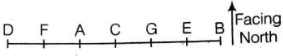Practice Mock Test for SNAP - 14 - Question 24

Direction: Seven persons A, B, C, D, E, F and G are sitting in a straight line (not necessarily in the same order) facing North. Only two persons sit between F and G and G sits second to the left of B.D sits third to the left of C. E sits exactly between G and B and B sits at the extreme right end of the row.

Q. Who amongst the following sits exactly in the middle of the line?

Detailed Solution for Practice Mock Test for SNAP - 14 - Question 24
The given information can be arranged asPractice Mock Test for SNAP - 14 - Question 25

Direction: Seven persons A, B, C, D, E, F and G are sitting in a straight line (not necessarily in the same order) facing North. Only two persons sit between F and G and G sits second to the left of B.D sits third to the left of C. E sits exactly between G and B and B sits at the extreme right end of the row

Q. What is the position of E with respect to A?

Detailed Solution for Practice Mock Test for SNAP - 14 - Question 25
The given information can be arranged asPractice Mock Test for SNAP - 14 - Question 26

If ‘A+ B’ means ‘A is the father of B’

If ‘AX B’ means ‘A is the sister of B’

If ‘A \$ B’ means ‘A is the wife of B’

If ‘A % B’ means ‘A is the mother of B’

If ‘A+ B’ means ‘A is the son of B’

Q. Which of the given expressions indicates that M is daughter of D?

Detailed Solution for Practice Mock Test for SNAP - 14 - Question 26
Here, we will try with each option.

From option (a)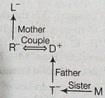Here, we do not know the gender of M, so relation cannot be established.

From option (b).

Practice Mock Test for SNAP - 14 - Question 27

Direction: Study the given information and answer the questions.

A word and number arrangement machine when given an input line of words and numbers rearranges them following a particular rule in each step. The following is an illustration of input and rearrangement. (Single digit numbers are preceded by a zero. All other numbers are two-digit numbers)

Input when 19 will you 07 he 40 coming 62 home 100 89

Step I be when 19 will you 07 40 coming 62 home 100 89

Step II be 07 when 19 will you 40 coming 62 home 100 89

Step III be 07 coming when 19 will you 40 62 home 100 89

Step IV be 07 coming 19 when will you 40 62 home 100 89

Step V be 07 coming 19 home when will you 40 62 100 89

Step VI be 07 coming 19 home 40 when will you 62 100 89

Step VII be 07 coming 19 home 40 when 62 will you 100 89

Step VIII be 07 coming 19 home 40 when 62 will 89 you 100

Step VIII is the last step of the arrangement of the above input as the intended arrangement is obtained. As per rules following in the above steps, find out in each of the questions the appropriate step for the given input.

Input next 57 problem 82 14 trend 02 purchase growth 41

Q. Which word/number would be the sixth position from the left end in Step III?

Detailed Solution for Practice Mock Test for SNAP - 14 - Question 27
In the given arrangement we observe that the word which has first alphabetical order in the dictionary comes first and next the number which is minimum among the input comes at second position. In the same manner, we arrange the following input. Finally, we get the arrangement in which number and letters are in increasing order.

In step III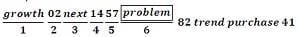The word ‘problem’ is at 6th position from the left end in step 41

Practice Mock Test for SNAP - 14 - Question 28

Direction: Study the given information and answer the questions.

A word and number arrangement machine when given an input line of words and numbers rearranges them following a particular rule in each step. The following is an illustration of input and rearrangement. (Single digit numbers are preceded by a zero. All other numbers are two-digit numbers)

Input when 19 will you 07 he 40 coming 62 home 100 89

Step I be when 19 will you 07 40 coming 62 home 100 89

Step II be 07 when 19 will you 40 coming 62 home 100 89

Step III be 07 coming when 19 will you 40 62 home 100 89

Step IV be 07 coming 19 when will you 40 62 home 100 89

Step V be 07 coming 19 home when will you 40 62 100 89

Step VI be 07 coming 19 home 40 when will you 62 100 89

Step VII be 07 coming 19 home 40 when 62 will you 100 89

Step VIII be 07 coming 19 home 40 when 62 will 89 you 100

Step VIII is the last step of the arrangement of the above input as the intended arrangement is obtained. As per rules following in the above steps, find out in each of the questions the appropriate step for the given input.

Input next 57 problem 82 14 trend 02 purchase growth 41

Q. Which word/number would be at position fifth from the right end in step III?

Detailed Solution for Practice Mock Test for SNAP - 14 - Question 28
In the given arrangement we observe that the word which has first alphabetical order in the dictionary comes first and next the number which is minimum among the input comes at second position. In the same manner, we arrange the following input. Finally, we get the arrangement in which number and letters are in increasing order.

Input just 14 and value 22 time 5 15Step Iand just 14 value 22 time 5 15

Step II and 5 just 14 value 22 time 15

Step III and 5 just 14 time value 22 15

Step IV and 5 just 14 time 15 value 22

Based on the above arrangement we answer the following questions. Step III and 5 just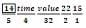‘14’ is at 5th position from the right end.

Practice Mock Test for SNAP - 14 - Question 29

Direction: Study the given information and answer the questions.

A word and number arrangement machine when given an input line of words and numbers rearranges them following a particular rule in each step. The following is an illustration of input and rearrangement. (Single digit numbers are preceded by a zero. All other numbers are two-digit numbers)

Input when 19 will you 07 he 40 coming 62 home 100 89

Step I be when 19 will you 07 40 coming 62 home 100 89

Step II be 07 when 19 will you 40 coming 62 home 100 89

Step III be 07 coming when 19 will you 40 62 home 100 89

Step IV be 07 coming 19 when will you 40 62 home 100 89

Step V be 07 coming 19 home when will you 40 62 100 89

Step VI be 07 coming 19 home 40 when will you 62 100 89

Step VII be 07 coming 19 home 40 when 62 will you 100 89

Step VIII be 07 coming 19 home 40 when 62 will 89 you 100

Step VIII is the last step of the arrangement of the above input as the intended arrangement is obtained. As per rules following in the above steps, find out in each of the questions the appropriate step for the given input.

Input next 57 problem 82 14 trend 02 purchase growth 41

Q. Which of the following would be Step II of the arrangement?

Detailed Solution for Practice Mock Test for SNAP - 14 - Question 29
In the given arrangement we observe that the word which has first alphabetical order in the dictionary comes first and next the number which is minimum among the input comes at second position. In the same manner, we arrange the following input. Finally, we get the arrangement in which number and letters are in increasing order.

Option (b) matches with step II of the arrangement

Practice Mock Test for SNAP - 14 - Question 30

Direction: Following questions are based on the five three digit numbers given below.

519 328 746 495 837

Q. If half of the second highest number is subtracted from the third highest number, then what will be the value?

Detailed Solution for Practice Mock Test for SNAP - 14 - Question 30
Third highest number = 519

Half of second highest number = 746/2 = 373

Thus, difference = 519 – 373 = 146

Practice Mock Test for SNAP - 14 - Question 31

Direction: Each of the questions below consists of a question and three statements numbered I and II given below it. You have to decide whether the data given in the statements are sufficient to answer the questions.

Q. There are six letters W, A, R, S, N and E. Is ‘ANSWER’ the word formed after performing the following operations using these six letters only?

I. E is placed fourth to the right o A. S is not placed immediately next to either A or E.

II. R is placed immediately next (either left or right) to E. W is placed immediately next (either left or right) to S.

III. Both N and W are placed immediately next to S. The word does not begin with R. A is not placed immediately next to W.

Detailed Solution for Practice Mock Test for SNAP - 14 - Question 31
Six letters W, A, R, S, N and E are given. We have to form the word ANSWER by performing operations given in statement.

In statement I

E is fourth to the right of A.

A _ _ _ E

1 2 3 4

S is not immediately next to either A or E.

A __ S __ E

In statement II

R is immediate next to E.

ER or R E

W is placed immediate next to S.

W S or S W

In statement III

Both N and W are immediate next to S.

N S W

A is not placed immediate next to W.

The word does not began with R.

Since, there are 6 alphabets in word ANSWER.

A _ S _ E _ (from Statement I)

ANS W E _ (from Statement III)

The word does not began with R and R is the only alphabet left. So, the word becomes ANSWER.

Therefore, Statement I and III are sufficient to answer the question.

Practice Mock Test for SNAP - 14 - Question 32

Direction: Each of the questions below consists of a question and three statements numbered I and II given below it. You have to decide whether the data given in the statements are sufficient to answer the questions.

Q. Point D is in which direction with respect to point B?

I. Point A is to the West of Point B. Point C is to the North of Point B. Point D is to the South of point C.

II. Point G is to the South of point D. point G is 4 m from point B. Point D is 9 m from point B

III. Point A is to the West of point B. Point B is exactly mid way between point A and E. Point F is to the South of point E. Point D is to the West of Point F.

Detailed Solution for Practice Mock Test for SNAP - 14 - Question 32
In Statement I

Point A is to the West of Point B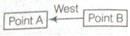Point C is to the North of Point B.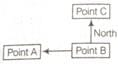Point D is to the South of point C.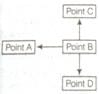In Statement II

Point G is to the South of point D.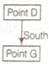Point G is 4 m from point B.

Here the direction is absent, so we cannot show this distance.

Point D is 9 m from point B

In this line also the direction is missing, so we cannot make the diagram. Therefore, data in Statement II are insufficient.

In Statement III

Point A is to the West of point B.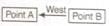Point B is exactly mid way between point A and E.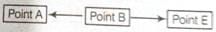Point F is to the South of point E.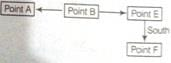Point D is to the West of Point F.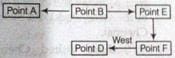Therefore, point D is to the South of point B. Hence, data in Statement I and III are sufficient to answer the question.

Practice Mock Test for SNAP - 14 - Question 33

Direction: In the following questions two/three statements are given followed by two conclusions numbered I and II. You have to take the three given statements to be true even, if they seem to be at variance from commonly known facts. Read both the conclusions and then decide which of the given conclusions logically follows from the given statements disregarding commonly known facts.

Q. Statements No fruit is a vegetable.

All potatoes are vegetables

Some fruits are apples.

Conclusions

I. No fruit is a potato.

II. Atleast some apples are fruits.

Detailed Solution for Practice Mock Test for SNAP - 14 - Question 33
All potatoes are vegetables + No vegetable is fruit = A + E = E = No potato is fruit conversion No fruit is a potato. Therefore, conclusion I follows. Again, Some fruits are apples (I) conversion (Atleast some apples are fruits (I). Therefore, conclusion II follows.)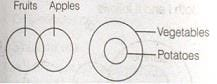So, both conclusions I and II follow.

Practice Mock Test for SNAP - 14 - Question 34

Direction: In the following questions two/three statements are given followed by two conclusions numbered I and II. You have to take the three given statements to be true even, if they seem to be at variance from commonly known facts. Read both the conclusions and then decide which of the given conclusions logically follows from the given statements disregarding commonly known facts.

Q. Statements All beans are pulses.

All pulses are crops.

No crops is a seed.

Conclusions

I. No seed is a bean.

II. No bean is a pulse.

Detailed Solution for Practice Mock Test for SNAP - 14 - Question 34
All beans are pulses + All pulses are crops + No crop is a seed = A + A + E = A + E = E = No bean is a seed conversion No seed is a bean. Therefore, conclusion I follows.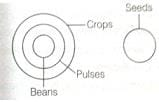So, only conclusion I follows.

Practice Mock Test for SNAP - 14 - Question 35

Direction: Study the given information carefully and answer the question.

Eight people E, F, G, H, W, X, Y and Z are sitting in two parallel rows containing four people each. E, F, G and H are sitting in row 1 facing North and W, X, Y and Z are sitting in row 2 facing South (but not necessarily in the same order)

Thus, each person sitting in row 1 faces another person sitting in row 2. Each of the two rows consists of one Doctor, one Engineer, one Pilot and one Scientist (but not necessarily in the same order)

• The Doctor of row 1 sits second to the right of H. X faces one of the immediate neighbours of H. Only one person sits between the X and the Scientist.

• The one who faces the Scientist of row 2 is an immediate neighbour of E. only one person sits between E and the Pilot.

• W sits second to the right of Z. Y does not face G. The Scientist of row 1 faces the Engineer of row 2.

• G faces one of the immediate neighbours of row 2. The Doctor of row 2 does not sit at any of the extreme ends of the line. Z is not a Doctor.

Which of the given statements is true with respect to the given arrangement?

Detailed Solution for Practice Mock Test for SNAP - 14 - Question 35
G is a scientist is true with respect to the given statement sol. : According to the given information, the sitting arrangement of eight people in two parallel rows is as shown below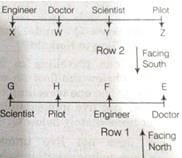Practice Mock Test for SNAP - 14 - Question 36

Direction: Study the given information carefully and answer the question.

Eight people E, F, G, H, W, X, Y and Z are sitting in two parallel rows containing four people each. E, F, G and H are sitting in row 1 facing North and W, X, Y and Z are sitting in row 2 facing South (but not necessarily in the same order)

Thus, each person sitting in row 1 faces another person sitting in row 2. Each of the two rows consists of one Doctor, one Engineer, one Pilot and one Scientist (but not necessarily in the same order)

• The Doctor of row 1 sits second to the right of H. X faces one of the immediate neighbours of H. Only one person sits between the X and the Scientist.

• The one who faces the Scientist of row 2 is an immediate neighbour of E. only one person sits between E and the Pilot.

• W sits second to the right of Z. Y does not face G. The Scientist of row 1 faces the Engineer of row 2.

• G faces one of the immediate neighbours of row 2. The Doctor of row 2 does not sit at any of the extreme ends of the line. Z is not a Doctor.

If Y and X interchange their places, so do H and Z, then who amongst the following not face E?

Detailed Solution for Practice Mock Test for SNAP - 14 - Question 36
If Y and X interchange their places, so do H and Z as shown below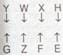So, E does not face F after interchanging the positions.

sol. : According to the given information, the sitting arrangement of eight people in two parallel rows is as shown belowPractice Mock Test for SNAP - 14 - Question 37

Direction: the questions below are given four statements followed by four conclusions numbered I, II, III and IV. You have to assume everything in the statements to be true even, if they seem to be at variance from commonly known facts and then decide which of the four conclusions logically follows from the given statements disregarding commonly known facts and select the appropriate answer.

Q. Statements Some numbers are digits.

All digits are alphabets

No alphabet is a vowel

All consonants are vowel.

Conculsion

I. No digit is a vowel

II. No alphabet is a consonant.

III. No vowel is a number

IV. All vowels are numbers

Detailed Solution for Practice Mock Test for SNAP - 14 - Question 37
All digits are alphabets (A) + No alphabet is vowel (E) = A + E = No digit is a vowel. Hence, conclusion I follows.

No alphabet is a vowel conversion No vowels is an alphabet.

Now, All consonants are vowels (A) + No vowel is an alphabet (E) = A + E = E = No consonant is an alphabet (E) conversion No alphabet is a consonant. Hence, conclusion II follows.

Now, III and IV are complementary pair.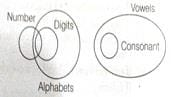Only conclusion I, II and either III or IV follow.

Practice Mock Test for SNAP - 14 - Question 38

Direction: In each question below a statement is given followed by two assumptions numbered I and II.

Q. Statement The employees association urged its members to stay away from the annual function as many of their demands were not met by the management.

Assumptions

I. Majority of the members of the association may not attend the function.

II. The management may cancel the annual function.

Detailed Solution for Practice Mock Test for SNAP - 14 - Question 38
Assumption I is implicit because members will follow association urge for their own interest. Assumption II is not implicit as the annual function will be held at a fixed date.
Practice Mock Test for SNAP - 14 - Question 39

Direction: In each question below a statement is given followed by two courses of action numbered I and II.

Q. Statement The police reported that two armed terrorists had entered the city a couple of days ago.

Courses of Action

I. Police should immediately circulate the photographs of the terrorists using electronic and print media in order to take public's help in nabbing them.

II. Public should be informed to stay indoors until the terrorists are arrested.

Detailed Solution for Practice Mock Test for SNAP - 14 - Question 39
Course of action I follows. This step will help police in nabbing the terrorist in a short period of time. Action II is impractical.
Practice Mock Test for SNAP - 14 - Question 40

Direction: In each question below a statement is given followed by two assumptions numbered I and II.

Q. Statement The highway police authority put up large boards at regular intervals indicating the speed limit and dangers of over speeding on the highways.

Assumptions

I. Most of the motorists may drive their vehicles within the speed limit on the highways.

II. Motorists. generally ignore such cautions and over speed on the highways.

Detailed Solution for Practice Mock Test for SNAP - 14 - Question 40
The notice has been given in the assumption that people will follow it and they will drive within the speed limit. So, assumption I is implicit. Assumption II is not implicit as it is a negative assumptions.
Practice Mock Test for SNAP - 14 - Question 41

If log2 [log3 (log2x)] = 1, then x is equal to

Detailed Solution for Practice Mock Test for SNAP - 14 - Question 41
log2 [log3 (log2 x)] = 1 = log2 2

→ log3 (log2 x) = 2

→ log2 x = 32 = 9

→ x = 29 = 512

Practice Mock Test for SNAP - 14 - Question 42

If α and β are the roots of the equation x2 – 2x + 4 = 0, then what is the value of α3 + β3?

Detailed Solution for Practice Mock Test for SNAP - 14 - Question 42
x2 – 2x + 4 = 0

α + β = 2; α . β = 4

α3 + β3 = (α + β) - 3αβ (α + β)

→ α3 + β3 8 – 3.4(2)

→ α3 + β3 = - 16

Practice Mock Test for SNAP - 14 - Question 43

The length of the longest rod that can be placed in a room which is 12 m long, 9 m broad and 8 m high is

Detailed Solution for Practice Mock Test for SNAP - 14 - Question 43
Required length = length of the diagonal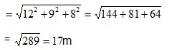Practice Mock Test for SNAP - 14 - Question 44

tan2 θ sin2θ is equal to

Detailed Solution for Practice Mock Test for SNAP - 14 - Question 44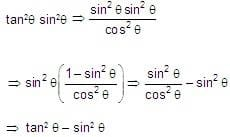Practice Mock Test for SNAP - 14 - Question 45

Probability of throwing 16 in one throw with three dice is

Detailed Solution for Practice Mock Test for SNAP - 14 - Question 45

The probability of throwing a specific number with one die is 1/6, since there are six possible outcomes and only one of them will be the number you are trying to throw. If you throw three dice at the same time, the probability of throwing a specific number with all three dice is (1/6)^3 = 1/216.

Therefore, the probability of throwing a 16 with three dice in one throw is 1/216.

Practice Mock Test for SNAP - 14 - Question 46

Direction: Each of the questions below consists of a question and two statements numbered (I) and (II) given below it. You have to decide whether the data provided in the statements are sufficient to answer the question.

Q. A person bought 2 kg of rice from a shop. But when he entered the next shop he found that the price was less. He calculated that if he bought 6 kg from that shop his average price would be Rs 20. Then what is the price in the first shop?

(I) The price in the second shop was Rs 18 per kg.

(II) The difference in the prices was Rs 8 per kg.

Detailed Solution for Practice Mock Test for SNAP - 14 - Question 46
Let the price in first shop = x per kg

(i)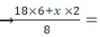20 → x can be determined.

(ii)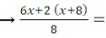20 → x can be determined.

∴ Each statement alone is sufficient to answer the question.

Practice Mock Test for SNAP - 14 - Question 47

If A (3, 5), B (-3, -4), C (7, 10) are the vertices of a the fourth vertex are?

Detailed Solution for Practice Mock Test for SNAP - 14 - Question 47
Midpoint of A (3, 5) and C (7, 10) = M(5, (15/2))

∴ Mild points of BD = M(5, (15/2))

B(-5, -4) and D(x, y)

∴(-5+x)/2 = 5, x = 10 + 5 = 15

(-4 + y)/2 = 15/2, y = 15 + 4 = 19

Co-ordinates of fourth vertex D = (15, 19)

Practice Mock Test for SNAP - 14 - Question 48

D, E, F are midpoints of BC, CA and AB respectively G.H. I are midpoints of FE, FD, DE respectively. Areas of ΔDHI and ΔAFE are in the ratio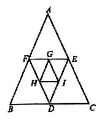Detailed Solution for Practice Mock Test for SNAP - 14 - Question 48
We have area of triangle AFE = A/4. (If A = Area of triangle ABC) and area of triangle DHI = (A/4)/4 = A/16.Hence, ratio = 1 : 4.
Practice Mock Test for SNAP - 14 - Question 49

A well 22.5 deep and of diameter 7 m has to be dug out. Find the cost of plastering its inner curved surface at Rs. 3 per sq. metre.

Detailed Solution for Practice Mock Test for SNAP - 14 - Question 49
Area of the inner curved surface of the well dug

=[2π x 3.5 x 22.5] = 2 x (22/7) x 3.5 x 22.5

= 44 x 0.5 x 22.5 = 495 sq.m.

∴ Total cost = 495 x 3 = Rs. 1485.

Practice Mock Test for SNAP - 14 - Question 50

Direction: Study the following graph carefully and answer the question given below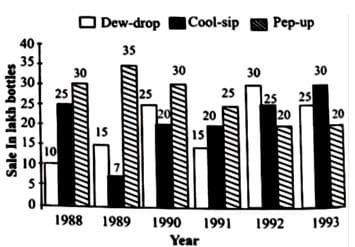In the case of Cool–sip drink, what was the approximate percent increase in sale in 1992 over its sale in 1991?

(i) Less than 20 (b) 20 –25

(ii) 25 (d) 31 – 35

(iii) 36 – 40

Detailed Solution for Practice Mock Test for SNAP - 14 - Question 50
Reqd. no. = ((25-20)/20) x 100 = 25%
Practice Mock Test for SNAP - 14 - Question 51

Two dice are thrown simultaneously then the probability of obtaining a total score of 5 is.

Detailed Solution for Practice Mock Test for SNAP - 14 - Question 51
Number of sample points on throwing two dice

= 6 x 6 = 36

The possible outcomes are (1, 4), (2, 3), (3, 2), (4, 1)

The probability of obtaining a total score of 5 is = 4/(6 x 6) = 1/9

Practice Mock Test for SNAP - 14 - Question 52

Which number should be added to 459045 to make it exactly divisible by 27?

Detailed Solution for Practice Mock Test for SNAP - 14 - Question 52
459045 is clearly divisible by 3.

∴ 459045 = 3 x 153015

& 153015 becomes divisible by 9 only after adding 3 into it.

∴ The number divisible by 27 is (153015 + 3) x 3 = 459045 + 9

Practice Mock Test for SNAP - 14 - Question 53

If p and q are the roots of the equation x2-px + q = 0, then what are the values of p and q respectively?

Detailed Solution for Practice Mock Test for SNAP - 14 - Question 53
p and q are roots

∴ They must satisfy equation

→ p2 – p(p) + q = 0

→ p2 – p2 + q = 0

→ q = 0

Also, q2 – pq + q = 0

→ q(q – p + 1) = 0

→ q = - 1 + p

→ p = q + 1

q = 0

∴ p = 1

Practice Mock Test for SNAP - 14 - Question 54

Number of bales of wool processed by 5 woollen mills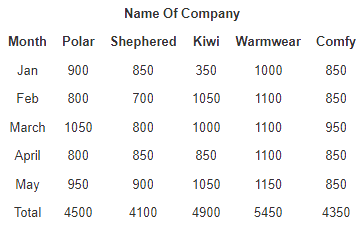Q. In the case of which mill is the processing of wool in March the highest percentage of the total processing by that mill during the five month period?

Detailed Solution for Practice Mock Test for SNAP - 14 - Question 54
Percentage processing of wool in the month of March by different companies.

Polar = 23.33%, Shepherd = 19.51%,

Kiwi = 20.41%, Warmwear = 20.18%

Comfy = 21.84%

Practice Mock Test for SNAP - 14 - Question 55

If nPr = 720 nCr, then r is equal to

Detailed Solution for Practice Mock Test for SNAP - 14 - Question 55
nPr = 720 nCrOr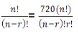→ r! = 720 = 1 x 2 x 3 x 4 x 5 x 6

Or r = 6

Practice Mock Test for SNAP - 14 - Question 56

The sum of two numbers is 25 and their difference is 13. Find their product.

Detailed Solution for Practice Mock Test for SNAP - 14 - Question 56
Let the numbers be x and y. Then, x + y = 25 and x – y = 13

→ x = 19, y = 6

→ xy = 114

Practice Mock Test for SNAP - 14 - Question 57

If four sides of a quadrilateral ABCD are tangential to a circle, then.

Detailed Solution for Practice Mock Test for SNAP - 14 - Question 57

Again AP, AQ are tangents to the circle from the point A.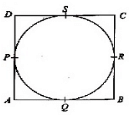AP = AQ

Similarly BR = BQ

CR = CS

DP = DS

(AP + DP) + (BR + CR) = AQ + DS + BQ + CS

= (AQ + BQ) + (CS + DS)

⇒ AD + BC = AB + CD

Practice Mock Test for SNAP - 14 - Question 58

tan 1° tan2° tan 3° .........tan89° is equal to

Detailed Solution for Practice Mock Test for SNAP - 14 - Question 58
tan 1° tan 2° tan 3° ....tan 89°

⇒ (tan 1°. tan 89°) (tan 2° tan 88°)....

.....(tan 44° tan 46°) tan 45°

⇒ (tan 1°. cot 1°) (tan 2° tan 2°)....

(tan 44° cot 44°) × 1

⇒ 1 × 1 × 1×.....× 1 = 1

Practice Mock Test for SNAP - 14 - Question 59

If nPr = nPr + 1 and nCr = nCr – 1, then the values of n and r are

Detailed Solution for Practice Mock Test for SNAP - 14 - Question 59
We have nPr = nPr+1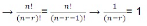Or n - r = 1 …(1)

Also, nCr - nCr-1 → r + r - 1 = n

→ 2r - n = 1 …(2)

Solving (1) and (2), we get r = 2 and n = 3

Practice Mock Test for SNAP - 14 - Question 60

Direction: Each of the question below consists of a question and two statements numbered (I) and (II) given below it. You have to decide whether the data provided in the statements are sufficient to answer the question.

Q. At present, how many villagers are voters in village ‘X’ ?

(I) There were 860 voters in village ‘X’ in the list prepared for the last election.

(II) The present list of village ‘X’ has 15% more voters than the list for the last election.

Detailed Solution for Practice Mock Test for SNAP - 14 - Question 60
(I) → No. of voters in the last election

(II) → No. of voters at present = 115% of no. of voters in the last election.

Both statements together are necessary to answer the question.

## Mock Test Series for SNAP

16 docs|33 tests
Information about Practice Mock Test for SNAP - 14 Page
In this test you can find the Exam questions for Practice Mock Test for SNAP - 14 solved & explained in the simplest way possible. Besides giving Questions and answers for Practice Mock Test for SNAP - 14, EduRev gives you an ample number of Online tests for practice

## Mock Test Series for SNAP

16 docs|33 tests

### How to Prepare for CAT

Read our guide to prepare for CAT which is created by Toppers & the best Teachers(Scan QR code)